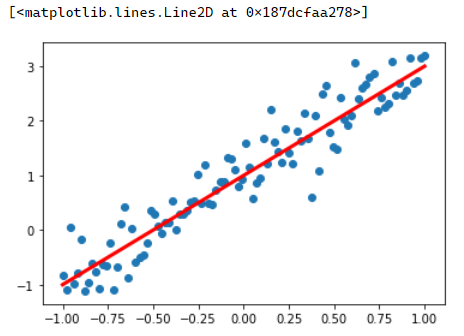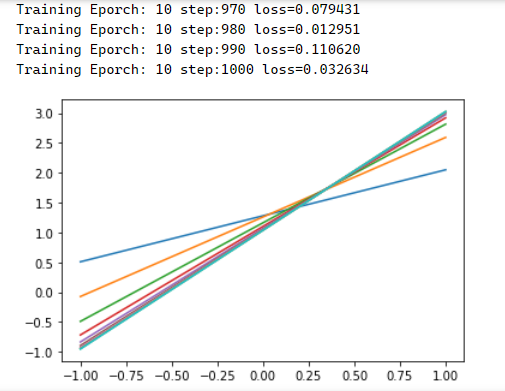Roger's

This is the way.

TensorFlow-2学习笔记-3-线性回归

### 实战练习

``````import tensorflow as tf
import numpy as np
import matplotlib.pyplot as plt
#在网页中使用matplotlib显示图像需要设置inline模式
%matplotlib inline
``````
``````x_data=np.linspace(-1,1,100)  # 等差数列生成100个在-1与1之间的点
np.random.seed(5)
y_data=2*x_data+1.0+np.random.randn(*x_data.shape)*0.4

#画出随机生成的样本的散点图
plt.scatter(x_data,y_data)
#画出想要通过学习得到的目标函数
plt.plot (x_data,1.0+2*x_data,'r',linewidth=3)
````````````def model(x,w,b):
return tf.multiply(x,w)+b
def loss(x,y,w,b):
err = model(x,w,b)-y  # 求差
squared_err = tf.square(err)  # 求平方得出方差
return tf.reduce_mean(squared_err)  # 求均方差
``````
``````w = tf.Variable(np.random.randn(),tf.float32)
b = tf.Variable(0.0,tf.float32)

training_epochs = 10 # 训练轮数
learning_rate = 0.01 # 学习率
``````
``````#计算梯度函数
loss_ = loss(x,y,w,b)
``````
``````# 执行训练
step = 0
loss_list = []
display_step = 10
for epoch in range(training_epochs):
for xs,ys in zip(x_data,y_data):
loss_=loss(xs,ys,w,b)
loss_list.append(loss_)

change_w = delta_w*learning_rate
change_b = delta_b*learning_rate
w.assign_sub(change_w)
b.assign_sub(change_b)

step=step+1
if step % display_step == 0:
print("Training Eporch:",'%02d' % (epoch+1),"step:%03d" % (step),
"loss=%.6f" % (loss_))
plt.plot(x_data,w.numpy()*x_data+b.numpy())
``````print("w:",w.numpy(),"b:",b.numpy()) 打印:w: 1.9897072 b: 1.0370641,看起来还是比较接近的

# 图形化查看损失变化情况

plt.plot(losslist) plt.plot(losslist,'r+')

``````x_test=3.21

predict=model(x_test,w.numpy(),b.numpy())
print("预测值:%f" % predict)

target = 2*x_test + 1.0
print("实际目标值:%f" % target)
``````

``````预测值:7.426406

``````

### 总结步骤

* 邮箱仅用于回复提醒，不会被公开